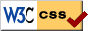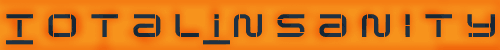﻿ T.i. Combat Robotics - Designing Around the Gyroscopic Effect

Designing Around the Gyroscopic Effect
Last updated - September 2006

DISCLAIMER: Combat robotics is a dangerous sport by it's nature. Extreme caution should be taken when performing any operation explained on this site. Any injury or death resulting from the use of these pages is the sole responsibility of the user and not totalinsanity.net. By undertaking the construction of a combat robot you assume all responsibility for your actions. When building a combat robot, always make sure you are, or are with, a responsible adult.

Build Responsibly

The gyroscopic effect is what causes robots with vertically spinning weapons to tip up when turning. This tutorial will describe how to design your robot so that your spinning weapon won't cause your robot to tip while turning.

If you don't wish to look over all the equations, go to the bottom of the page for a calculator that will do the math for you.

There is actually a very simple formula to figure out what the forces are:

M = I * w1 * w2

• I is the mass moment of inertia of the spinning weapon system, measured in Kilogram*meters^2 Click Here to find the mass moment of inertia for your weapon.

• w1 and w2 are the rotational velocities of the weapon and the turning robot in the units of radians/second. It does not matter which label you use for which speed. To convert the RPM of your weapon to radians/second, use this formula: w = (RPM * Pi) / 30

• M is the resulting moment applied to the chassis measured in Newton*meters. With a standard vertically spinning weapon, when turning left, the right wheel will raise. When turning right, the left wheel will raise.

To figure out how fast the robot is rotating when you have one side of your drive in forward, and one in reverse(maximum turning), use this formula:

w = (RPM * D * Pi) / (30 * L)

• L is the width of your robot's drive train.

• D is the diameter of your wheels.

• RPM is the RPM of your drive motors.

• w is the how fast your robot is rotating, in radians/second.

You don't need to use meters for length here, but make sure that L and D are in the same units, they will cancel.

The ideal robot, when turning at max speed, will not have either of it's wheels raise. To figure out if your robot's wheels will lift when turning, use the following formula:

tip = M/[( L^2) * Weight]

• Weight is the weight of your robot in Newtons (kilograms*9.8).

• L must be in meters.

• If you will turn by simply stopping one wheel while powering the other, divide tip by 2.

If tip is >1, the robot will raise a wheel when turning. If tip stays <1, your robot will never have a wheel lift, but if tip is near 1 one side of your drive train will have little traction when turning sharply, slowing your turn.

Tip should be minimized. The easiest way to minimize this number is to increase the width of your robot (notice that it is squared in the denominator of the equation).

To figure out the force on the raising wheel (this would normally be a positive number equal to half your robots weight) use this formula:

force = (Weight * L/2) - M/2

If this force is negative the wheel will lift. If the arena surface is magnetic, the lifting force may be countered by chassis magnets located near the wheels. The magnetic force must equal or exceed the gyroscopic lifting force to be effective.

Now that you know how to do it by hand, here's a calculator to save you the trouble...

Gyroscopic Effect Calculator (requires javascript)
If you need help converting units, download this program: Convert

 Drive Train Width mm Drive Motor Speed RPM Wheel Diameter mm Robot Mass kilograms Weapon Mass Moment of Inertia kg·m² Weapon Speed RPM Max Robot Spin Rate Second per revolution Force on Raising Wheel Newtons @ Max Spin Rate Max Flat Turn Rate Second per revolution

'Max Robot Spin Rate' is the quickest the robot can rotate 360 degrees, spining in place with negligable turning friction.

'Force on Raising Wheel' with no gyro effect would be half the weight of your robot.

• If the value is positive the robot can spin at full speed without wheel lift.
• If the value is close to zero one wheel will have very little traction in a high-rate turn.
• If the value is negative one wheel may lift off the floor in a full-speed spin.

'Max Flat Turn Rate' is the quickest the robot is able to rotate 360 degrees without wheel lift. You may restrict the turning rate of your robot by setting a 'Dual Rate', 'ATV', or 'Travel Adjust' on your R/C transmitter. Consult your radio manual for details.

For a more in depth description of the gyro effect, visit: http://www.gyroscopes.org/math.asp© 2006 by T.i. Combat Robotics unless otherwise noted.c o m b a t - r o b o t i c s